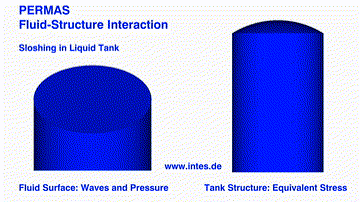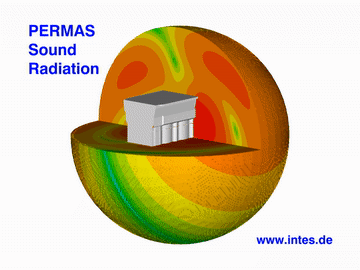# Fluid-Structure Acoustics

The PERMAS-FS (Fluid-Structure Acoustics) module allows for the calculation of acoustic modes, as well as eigenvalues of coupled fluid-structure systems and the computation of coupled or uncoupled response in the frequency or time domain. This computation is provided either directly or in modal coordinates.

The fluid may be compressible or incompressible. All fluid absorption or damping properties are also available for the uncoupled computation. The fluid damping may be frequency dependent.

A number of special features is provided to model boundary conditions:

• Surface waves are modeled by specific elements.
• Special coupling elements are provided at the boundary of the fluid to the structural model. These elements are also used to model surface absorption. In addition, another acoustic damping facility is available through volumetric dampers (like seats in a car).
• Semi-infinite elements are provided to handle an infinite surrounding space.
• Radiating boundary conditions (RBC) can be modeled using special element families, one following the theory of Bayliss-Turkel and another the theory of Engquist-Madya.

For the coupling elements mentioned above the face normal has to be oriented from the fluid to the structure. This condition is checked automatically in order to avoid conflicts and sources of mistakes in the coupling of fluid and structure.

For the calculation of dynamic mode frequencies, a difference is made between the fully coupled modes and the structural modes modified by the additional fluid mass:

• The calculation of real eigenvalues and mode shapes of the coupled structure is realized by a simultaneous vector iteration. The specification of a number of modes and an upper frequency limit is supported. The special formulation of the algorithm used is capable of solving very large eigenvalue problems in an efficient way.
• The added mass problem can be solved with the standard structural eigenvalue solver where the mass of the fluid is taken into account to calculate the structural modes.

The runtime for a coupled eigenvalue analysis in case of large models with a high number of modes can be drastically reduced by the MLDR method.

For the calculation of the dynamic response behavior, the following methods are available:

• The response in the time domain (transient response) is determined by a modal solution of the equation of motion. Available solvers are Newmark β; or HHT (Hilber-Hughes-Taylor)
• The response in the frequency domain (frequency response) is determined by the modal or direct solution of the linear complex system of equations for each excitation frequency requested. In general, a fully populated equation system has to be solved. In modal space an iterative solver makes the analysis much faster.

By specification of a node and/or element set the run time for modal superposition methods can be drastically reduced when the results are only determined for the set members. The reduced response results in enormous disk space savings.

The following damping features are available:
• For the structure:
material or structural damping for elements, also frequency dependent, if required.
-global structral damping
-Rayleigh damping
• For the fluid:
- boundary absorption,
- volumetric absorption (also frequency-dependent).
• For the coupled system:
modal viscous damping (coupled system).

The excitation is defined by static loading cases modulated by functions of frequency. The load definitions may consist of:

• Structural loads as described for the DRA module
• prescribed pressure.

Results
The primary results are:
• displacements,
• pressure,
• velocities, and
• accelerations.

For the drawing of xy-plots, these data may be output as a function of time respectively frequency. Moreover, the following results may be derived:

• reaction forces,
• stresses and stress resultants,
• strain energy,
• kinetic energy,
• specific sound radiation power density,
• sound particle velocity.Sloshing in liquid tanks during earthquake.Sound radiation of an engine (sound pressure distribution).